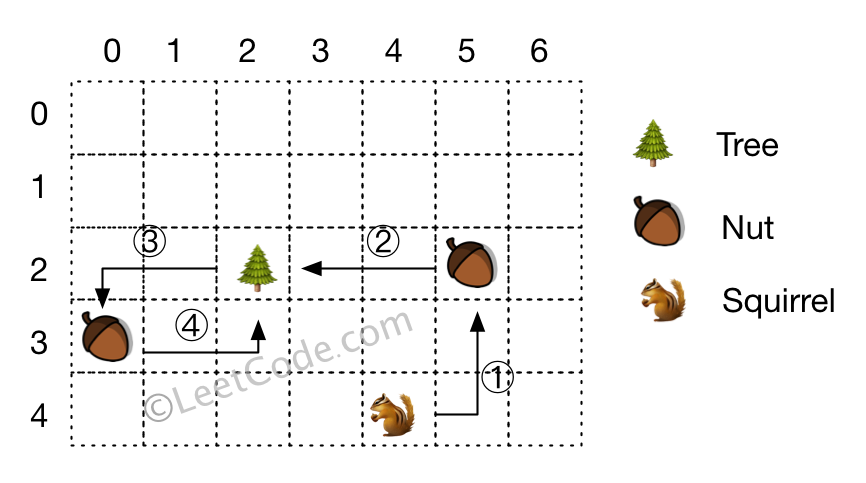# LeetCode: 573. 松鼠模拟¶

## 1、题目描述¶

输入:• 所有给定的位置不会重叠。
• 松鼠一次最多只能携带一颗坚果。
• 给定的坚果位置没有顺序。
• 高度和宽度是正整数。 3 <= 高度 * 宽度 <= 10,000
• 给定的网格至少包含一颗坚果，唯一的一棵树和一只松鼠。

## 2、解题思路¶

• 松鼠从初始位置先到一个坚果的位置，然后回到树
• 剩余的坚果都是从树上去，然后回到树上，就是2倍的曼哈顿距离
• 只需要找到第一步，也就是松鼠先去拿的坚果，然后回到树上这一步所有的距离对整体的步数减少效果最明显的一个即可
class Solution:
def minDistance(self, height: int, width: int, tree: List[int], squirrel: List[int], nuts: List[List[int]]) -> int:
length = len(nuts)
tree_length = abs(tree - nuts) + abs(tree - nuts)
squirrel_length = abs(squirrel - nuts) + abs(squirrel - nuts)
save_path = [tree_length - squirrel_length, tree_length, squirrel_length]
ans = tree_length + squirrel_length
for i in range(1, length):
cur_tree_length = abs(tree - nuts[i]) + abs(tree - nuts[i])
cur_squirrel_length = abs(squirrel - nuts[i]) + abs(squirrel - nuts[i])
if cur_tree_length - cur_squirrel_length > save_path:
ans -= save_path
ans += save_path
ans += cur_tree_length + cur_squirrel_length
save_path = [cur_tree_length - cur_squirrel_length, cur_tree_length, cur_squirrel_length]
else:
ans += cur_tree_length * 2
return ans